## Classification Using Nearest Neighbors

### Pairwise Distance Metrics

Categorizing query points based on their distance to points in a training data set can be a simple yet effective way of classifying new points. You can use various metrics to determine the distance, described next. Use `pdist2` to find the distance between a set of data and query points.

#### Distance Metrics

Given an mx-by-n data matrix X, which is treated as mx (1-by-n) row vectors x1, x2, ..., xmx, and an my-by-n data matrix Y, which is treated as my (1-by-n) row vectors y1, y2, ...,ymy, the various distances between the vector xs and yt are defined as follows:

• Euclidean distance

`${d}_{st}^{2}=\left({x}_{s}-{y}_{t}\right)\left({x}_{s}-{y}_{t}{\right)}^{\prime }.$`

The Euclidean distance is a special case of the Minkowski distance, where p = 2.

• Standardized Euclidean distance

`${d}_{st}^{2}=\left({x}_{s}-{y}_{t}\right){V}^{-1}\left({x}_{s}-{y}_{t}{\right)}^{\prime },$`

where V is the n-by-n diagonal matrix whose jth diagonal element is (S(j))2, where S is a vector of scaling factors for each dimension.

• Mahalanobis distance

`${d}_{st}^{2}=\left({x}_{s}-{y}_{t}\right){C}^{-1}\left({x}_{s}-{y}_{t}{\right)}^{\prime },$`

where C is the covariance matrix.

• City block distance

`${d}_{st}=\sum _{j=1}^{n}|{x}_{sj}-{y}_{tj}|.$`

The city block distance is a special case of the Minkowski distance, where p = 1.

• Minkowski distance

`${d}_{st}=\sqrt[p]{\sum _{j=1}^{n}{|{x}_{sj}-{y}_{tj}|}^{p}}.$`

For the special case of p = 1, the Minkowski distance gives the city block distance. For the special case of p = 2, the Minkowski distance gives the Euclidean distance. For the special case of p = ∞, the Minkowski distance gives the Chebychev distance.

• Chebychev distance

`${d}_{st}={\mathrm{max}}_{j}\left\{|{x}_{sj}-{y}_{tj}|\right\}.$`

The Chebychev distance is a special case of the Minkowski distance, where p = ∞.

• Cosine distance

`${d}_{st}=\left(1-\frac{{x}_{s}{{y}^{\prime }}_{t}}{\sqrt{\left({x}_{s}{{x}^{\prime }}_{s}\right)\left({y}_{t}{{y}^{\prime }}_{t}\right)}}\right).$`
• Correlation distance

`${d}_{st}=1-\frac{\left({x}_{s}-{\overline{x}}_{s}\right){\left({y}_{t}-{\overline{y}}_{t}\right)}^{\prime }}{\sqrt{\left({x}_{s}-{\overline{x}}_{s}\right){\left({x}_{s}-{\overline{x}}_{s}\right)}^{\prime }}\sqrt{\left({y}_{t}-{\overline{y}}_{t}\right){\left({y}_{t}-{\overline{y}}_{t}\right)}^{\prime }}},$`

where

`${\overline{x}}_{s}=\frac{1}{n}\sum _{j}{x}_{sj}$`

and

`${\overline{y}}_{t}=\frac{1}{n}\sum _{j}{y}_{tj}.$`
• Hamming distance

`${d}_{st}=\left(#\left({x}_{sj}\ne {y}_{tj}\right)/n\right).$`
• Jaccard distance

`${d}_{st}=\frac{#\left[\left({x}_{sj}\ne {y}_{tj}\right)\cap \left(\left({x}_{sj}\ne 0\right)\cup \left({y}_{tj}\ne 0\right)\right)\right]}{#\left[\left({x}_{sj}\ne 0\right)\cup \left({y}_{tj}\ne 0\right)\right]}.$`
• Spearman distance

`${d}_{st}=1-\frac{\left({r}_{s}-{\overline{r}}_{s}\right){\left({r}_{t}-{\overline{r}}_{t}\right)}^{\prime }}{\sqrt{\left({r}_{s}-{\overline{r}}_{s}\right){\left({r}_{s}-{\overline{r}}_{s}\right)}^{\prime }}\sqrt{\left({r}_{t}-{\overline{r}}_{t}\right){\left({r}_{t}-{\overline{r}}_{t}\right)}^{\prime }}},$`

where

• rsj is the rank of xsj taken over x1j, x2j, ...xmx,j, as computed by `tiedrank`.

• rtj is the rank of ytj taken over y1j, y2j, ...ymy,j, as computed by `tiedrank`.

• rs and rt are the coordinate-wise rank vectors of xs and yt, that is, rs = (rs1, rs2, ... rsn) and rt = (rt1, rt2, ... rtn).

• ${\overline{r}}_{s}=\frac{1}{n}\sum _{j}{r}_{sj}=\frac{\left(n+1\right)}{2}$.

• ${\overline{r}}_{t}=\frac{1}{n}\sum _{j}{r}_{tj}=\frac{\left(n+1\right)}{2}$.

### k-Nearest Neighbor Search and Radius Search

Given a set X of n points and a distance function, k-nearest neighbor (kNN) search lets you find the k closest points in X to a query point or set of points `Y`. The kNN search technique and kNN-based algorithms are widely used as benchmark learning rules. The relative simplicity of the kNN search technique makes it easy to compare the results from other classification techniques to kNN results. The technique has been used in various areas such as:

• bioinformatics

• image processing and data compression

• document retrieval

• computer vision

• multimedia database

• marketing data analysis

You can use kNN search for other machine learning algorithms, such as:

• kNN classification

• local weighted regression

• missing data imputation and interpolation

• density estimation

You can also use kNN search with many distance-based learning functions, such as K-means clustering.

In contrast, for a positive real value `r`, `rangesearch` finds all points in `X` that are within a distance `r` of each point in `Y`. This fixed-radius search is closely related to kNN search, as it supports the same distance metrics and search classes, and uses the same search algorithms.

#### k-Nearest Neighbor Search Using Exhaustive Search

When your input data meets any of the following criteria, `knnsearch` uses the exhaustive search method by default to find the k-nearest neighbors:

• The number of columns of `X` is more than 10.

• `X` is sparse.

• The distance metric is either:

• `'seuclidean'`

• `'mahalanobis'`

• `'cosine'`

• `'correlation'`

• `'spearman'`

• `'hamming'`

• `'jaccard'`

• A custom distance function

`knnsearch` also uses the exhaustive search method if your search object is an `ExhaustiveSearcher` model object. The exhaustive search method finds the distance from each query point to every point in `X`, ranks them in ascending order, and returns the k points with the smallest distances. For example, this diagram shows the k = 3 nearest neighbors.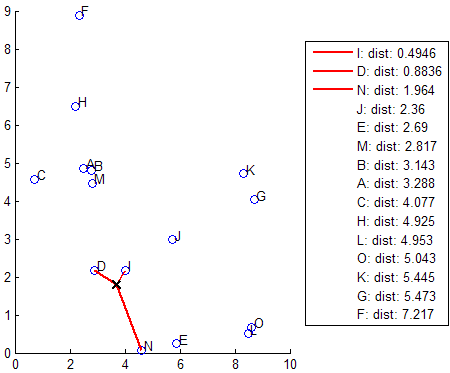#### k-Nearest Neighbor Search Using a Kd-Tree

When your input data meets all of the following criteria, `knnsearch` creates a Kd-tree by default to find the k-nearest neighbors:

• The number of columns of `X` is less than 10.

• `X` is not sparse.

• The distance metric is either:

• `'euclidean'` (default)

• `'cityblock'`

• `'minkowski'`

• `'chebychev'`

`knnsearch` also uses a Kd-tree if your search object is a `KDTreeSearcher` model object.

Kd-trees divide your data into nodes with at most `BucketSize` (default is 50) points per node, based on coordinates (as opposed to categories). The following diagrams illustrate this concept using `patch` objects to color code the different “buckets.”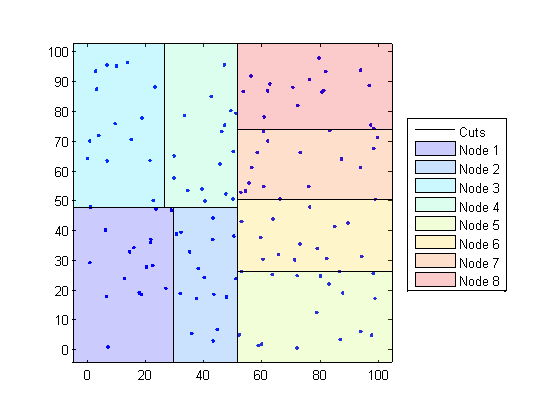When you want to find the k-nearest neighbors to a given query point, `knnsearch` does the following:

1. Determines the node to which the query point belongs. In the following example, the query point (32,90) belongs to Node 4.

2. Finds the closest k points within that node and its distance to the query point. In the following example, the points in red circles are equidistant from the query point, and are the closest points to the query point within Node 4.

3. Chooses all other nodes having any area that is within the same distance, in any direction, from the query point to the kth closest point. In this example, only Node 3 overlaps the solid black circle centered at the query point with radius equal to the distance to the closest points within Node 4.

4. Searches nodes within that range for any points closer to the query point. In the following example, the point in a red square is slightly closer to the query point than those within Node 4.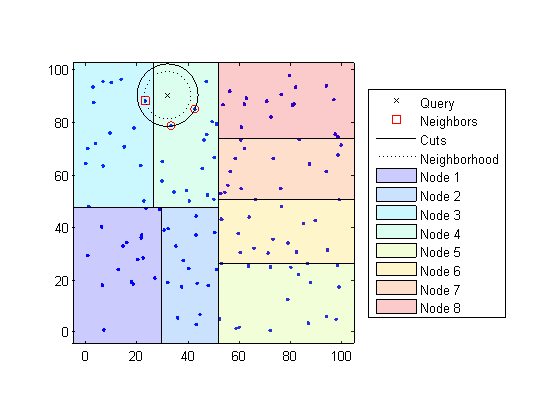Using a Kd-tree for large data sets with fewer than 10 dimensions (columns) can be much more efficient than using the exhaustive search method, as `knnsearch` needs to calculate only a subset of the distances. To maximize the efficiency of Kd-trees, use a `KDTreeSearcher` model.

#### What Are Search Model Objects?

Basically, model objects are a convenient way of storing information. Related models have the same properties with values and types relevant to a specified search method. In addition to storing information within models, you can perform certain actions on models.

You can efficiently perform a k-nearest neighbors search on your search model using `knnsearch`. Or, you can search for all neighbors within a specified radius using your search model and `rangesearch`. In addition, there are a generic `knnsearch` and `rangesearch` functions that search without creating or using a model.

To determine which type of model and search method is best for your data, consider the following:

• Does your data have many columns, say more than 10? The `ExhaustiveSearcher` model may perform better.

• Is your data sparse? Use the `ExhaustiveSearcher` model.

• Do you want to use one of these distance metrics to find the nearest neighbors? Use the `ExhaustiveSearcher` model.

• `'seuclidean'`

• `'mahalanobis'`

• `'cosine'`

• `'correlation'`

• `'spearman'`

• `'hamming'`

• `'jaccard'`

• A custom distance function

• Is your data set huge (but with fewer than 10 columns)? Use the `KDTreeSearcher` model.

• Are you searching for the nearest neighbors for a large number of query points? Use the `KDTreeSearcher` model.

### Classify Query Data

This example shows how to classify query data by:

1. Growing a Kd-tree

2. Conducting a k nearest neighbor search using the grown tree.

3. Assigning each query point the class with the highest representation among their respective nearest neighbors.

Classify a new point based on the last two columns of the Fisher iris data. Using only the last two columns makes it easier to plot.

```load fisheriris x = meas(:,3:4); gscatter(x(:,1),x(:,2),species) legend('Location','best')```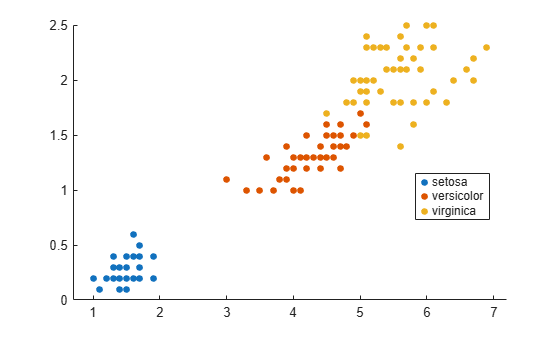Plot the new point.

```newpoint = [5 1.45]; line(newpoint(1),newpoint(2),'marker','x','color','k',... 'markersize',10,'linewidth',2)```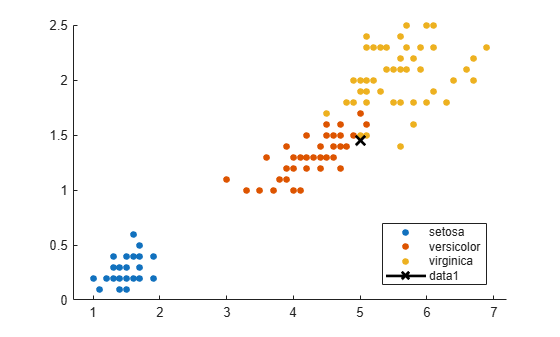Prepare a Kd-tree neighbor searcher model.

`Mdl = KDTreeSearcher(x)`
```Mdl = KDTreeSearcher with properties: BucketSize: 50 Distance: 'euclidean' DistParameter: [] X: [150x2 double] ```

`Mdl` is a `KDTreeSearcher` model. By default, the distance metric it uses to search for neighbors is Euclidean distance.

Find the 10 sample points closest to the new point.

```[n,d] = knnsearch(Mdl,newpoint,'k',10); line(x(n,1),x(n,2),'color',[.5 .5 .5],'marker','o',... 'linestyle','none','markersize',10)```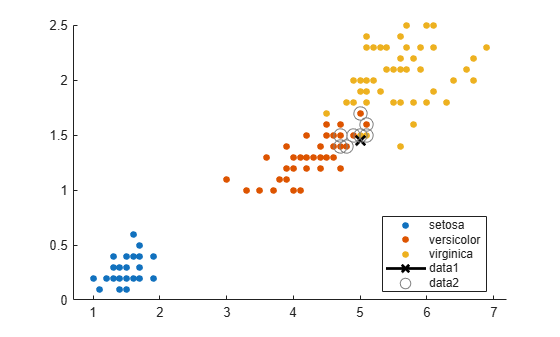It appears that `knnsearch` has found only the nearest eight neighbors. In fact, this particular dataset contains duplicate values.

`x(n,:)`
```ans = 10×2 5.0000 1.5000 4.9000 1.5000 4.9000 1.5000 5.1000 1.5000 5.1000 1.6000 4.8000 1.4000 5.0000 1.7000 4.7000 1.4000 4.7000 1.4000 4.7000 1.5000 ```

Make the axes equal so the calculated distances correspond to the apparent distances on the plot axis equal and zoom in to see the neighbors better.

```xlim([4.5 5.5]); ylim([1 2]); axis square```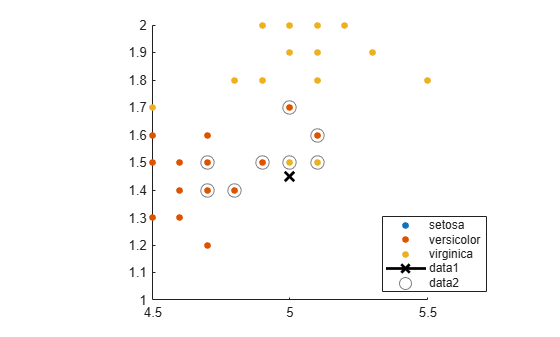Find the species of the 10 neighbors.

`tabulate(species(n))`
``` Value Count Percent virginica 2 20.00% versicolor 8 80.00% ```

Using a rule based on the majority vote of the 10 nearest neighbors, you can classify this new point as a versicolor.

Visually identify the neighbors by drawing a circle around the group of them. Define the center and diameter of a circle, based on the location of the new point.

```ctr = newpoint - d(end); diameter = 2*d(end); % Draw a circle around the 10 nearest neighbors. h = rectangle('position',[ctr,diameter,diameter],... 'curvature',[1 1]); h.LineStyle = ':';```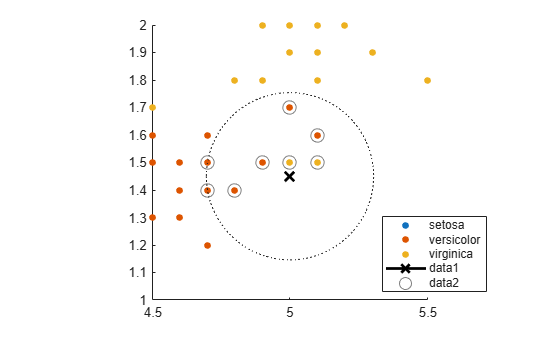Using the same dataset, find the 10 nearest neighbors to three new points.

```figure newpoint2 = [5 1.45;6 2;2.75 .75]; gscatter(x(:,1),x(:,2),species) legend('location','best') [n2,d2] = knnsearch(Mdl,newpoint2,'k',10); line(x(n2,1),x(n2,2),'color',[.5 .5 .5],'marker','o',... 'linestyle','none','markersize',10) line(newpoint2(:,1),newpoint2(:,2),'marker','x','color','k',... 'markersize',10,'linewidth',2,'linestyle','none')```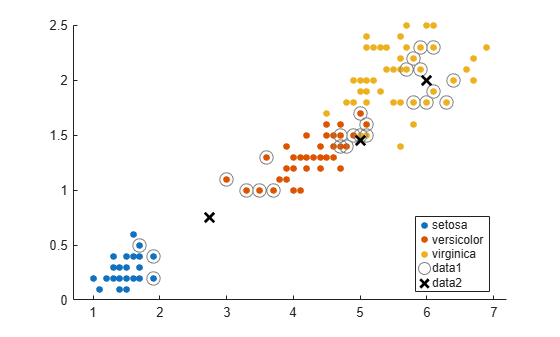Find the species of the 10 nearest neighbors for each new point.

`tabulate(species(n2(1,:)))`
``` Value Count Percent virginica 2 20.00% versicolor 8 80.00% ```
`tabulate(species(n2(2,:)))`
``` Value Count Percent virginica 10 100.00% ```
`tabulate(species(n2(3,:)))`
``` Value Count Percent versicolor 7 70.00% setosa 3 30.00% ```

For more examples using `knnsearch` methods and function, see the individual reference pages.

### Find Nearest Neighbors Using a Custom Distance Metric

This example shows how to find the indices of the three nearest observations in `X` to each observation in `Y` with respect to the chi-square distance. This distance metric is used in correspondence analysis, particularly in ecological applications.

Randomly generate normally distributed data into two matrices. The number of rows can vary, but the number of columns must be equal. This example uses 2-D data for plotting.

```rng(1); % For reproducibility X = randn(50,2); Y = randn(4,2); h = zeros(3,1); figure; h(1) = plot(X(:,1),X(:,2),'bx'); hold on; h(2) = plot(Y(:,1),Y(:,2),'rs','MarkerSize',10); title('Heterogenous Data')```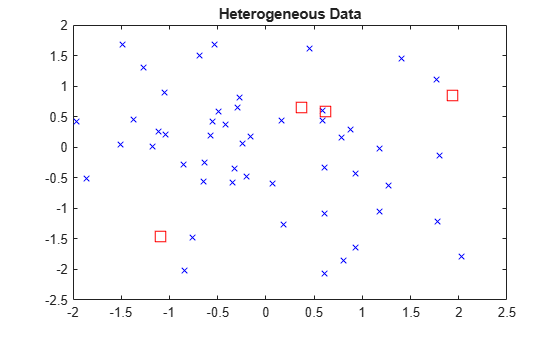The rows of `X` and `Y` correspond to observations, and the columns are, in general, dimensions (for example, predictors).

The chi-square distance between j-dimensional points x and z is

`$\chi \left(x,z\right)=\sqrt{\sum _{j=1}^{J}{w}_{j}{\left({x}_{j}-{z}_{j}\right)}^{2}},$`

where ${w}_{j}$ is the weight associated with dimension j.

Choose weights for each dimension, and specify the chi-square distance function. The distance function must:

• Take as input arguments one row of `X`, e.g., `x`, and the matrix `Z`.

• Compare `x` to each row of `Z`.

• Return a vector `D` of length ${n}_{z}$, where ${n}_{z}$ is the number of rows of `Z`. Each element of `D` is the distance between the observation corresponding to `x` and the observations corresponding to each row of `Z`.

```w = [0.4; 0.6]; chiSqrDist = @(x,Z)sqrt((bsxfun(@minus,x,Z).^2)*w);```

This example uses arbitrary weights for illustration.

Find the indices of the three nearest observations in `X` to each observation in `Y`.

```k = 3; [Idx,D] = knnsearch(X,Y,'Distance',chiSqrDist,'k',k);```

`idx` and `D` are 4-by-3 matrices.

• `idx(j,1)` is the row index of the closest observation in `X` to observation j of `Y`, and `D(j,1)` is their distance.

• `idx(j,2)` is the row index of the next closest observation in `X` to observation j of `Y`, and `D(j,2)` is their distance.

• And so on.

Identify the nearest observations in the plot.

```for j = 1:k; h(3) = plot(X(Idx(:,j),1),X(Idx(:,j),2),'ko','MarkerSize',10); end legend(h,{'\texttt{X}','\texttt{Y}','Nearest Neighbor'},'Interpreter','latex'); title('Heterogenous Data and Nearest Neighbors') hold off;```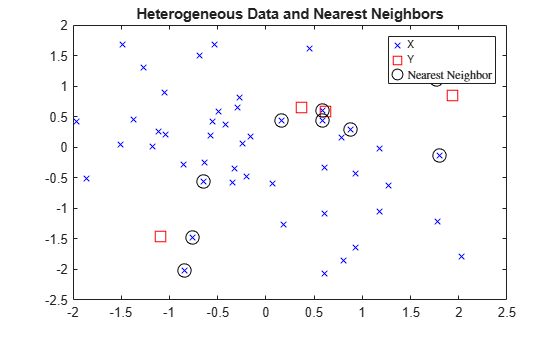Several observations of `Y` share nearest neighbors.

Verify that the chi-square distance metric is equivalent to the Euclidean distance metric, but with an optional scaling parameter.

```[IdxE,DE] = knnsearch(X,Y,'Distance','seuclidean','k',k,... 'Scale',1./(sqrt(w))); AreDiffIdx = sum(sum(Idx ~= IdxE))```
```AreDiffIdx = 0 ```
`AreDiffDist = sum(sum(abs(D - DE) > eps))`
```AreDiffDist = 0 ```

The indices and distances between the two implementations of three nearest neighbors are practically equivalent.

### K-Nearest Neighbor Classification for Supervised Learning

The `ClassificationKNN` classification model lets you:

Prepare your data for classification according to the procedure in Steps in Supervised Learning. Then, construct the classifier using `fitcknn`.

### Construct KNN Classifier

This example shows how to construct a k-nearest neighbor classifier for the Fisher iris data.

```load fisheriris X = meas; % Use all data for fitting Y = species; % Response data```

Construct the classifier using `fitcknn`.

`Mdl = fitcknn(X,Y)`
```Mdl = ClassificationKNN ResponseName: 'Y' CategoricalPredictors: [] ClassNames: {'setosa' 'versicolor' 'virginica'} ScoreTransform: 'none' NumObservations: 150 Distance: 'euclidean' NumNeighbors: 1 Properties, Methods ```

A default k-nearest neighbor classifier uses a single nearest neighbor only. Often, a classifier is more robust with more neighbors than that.

Change the neighborhood size of `Mdl` to `4`, meaning that `Mdl` classifies using the four nearest neighbors.

`Mdl.NumNeighbors = 4;`

### Examine Quality of KNN Classifier

This example shows how to examine the quality of a k-nearest neighbor classifier using resubstitution and cross validation.

Construct a KNN classifier for the Fisher iris data as in Construct KNN Classifier.

```load fisheriris X = meas; Y = species; rng(10); % For reproducibility Mdl = fitcknn(X,Y,'NumNeighbors',4);```

Examine the resubstitution loss, which, by default, is the fraction of misclassifications from the predictions of `Mdl`. (For nondefault cost, weights, or priors, see `loss`.).

`rloss = resubLoss(Mdl)`
```rloss = 0.0400 ```

The classifier predicts incorrectly for 4% of the training data.

Construct a cross-validated classifier from the model.

`CVMdl = crossval(Mdl);`

Examine the cross-validation loss, which is the average loss of each cross-validation model when predicting on data that is not used for training.

`kloss = kfoldLoss(CVMdl)`
```kloss = 0.0333 ```

The cross-validated classification accuracy resembles the resubstitution accuracy. Therefore, you can expect `Mdl` to misclassify approximately 4% of new data, assuming that the new data has about the same distribution as the training data.

### Predict Classification Using KNN Classifier

This example shows how to predict classification for a k-nearest neighbor classifier.

Construct a KNN classifier for the Fisher iris data as in Construct KNN Classifier.

```load fisheriris X = meas; Y = species; Mdl = fitcknn(X,Y,'NumNeighbors',4);```

Predict the classification of an average flower.

```flwr = mean(X); % an average flower flwrClass = predict(Mdl,flwr)```
```flwrClass = 1x1 cell array {'versicolor'} ```

### Modify KNN Classifier

This example shows how to modify a k-nearest neighbor classifier.

Construct a KNN classifier for the Fisher iris data as in Construct KNN Classifier.

```load fisheriris X = meas; Y = species; Mdl = fitcknn(X,Y,'NumNeighbors',4);```

Modify the model to use the three nearest neighbors, rather than the default one nearest neighbor.

`Mdl.NumNeighbors = 3;`

Compare the resubstitution predictions and cross-validation loss with the new number of neighbors.

`loss = resubLoss(Mdl)`
```loss = 0.0400 ```
```rng(10); % For reproducibility CVMdl = crossval(Mdl,'KFold',5); kloss = kfoldLoss(CVMdl)```
```kloss = 0.0333 ```

In this case, the model with three neighbors has the same cross-validated loss as the model with four neighbors (see Examine Quality of KNN Classifier).

Modify the model to use cosine distance instead of the default, and examine the loss. To use cosine distance, you must recreate the model using the exhaustive search method.

```CMdl = fitcknn(X,Y,'NSMethod','exhaustive','Distance','cosine'); CMdl.NumNeighbors = 3; closs = resubLoss(CMdl)```
```closs = 0.0200 ```

The classifier now has lower resubstitution error than before.

Check the quality of a cross-validated version of the new model.

```CVCMdl = crossval(CMdl); kcloss = kfoldLoss(CVCMdl)```
```kcloss = 0.0200 ```

`CVCMdl` has a better cross-validated loss than `CVMdl`. However, in general, improving the resubstitution error does not necessarily produce a model with better test-sample predictions.